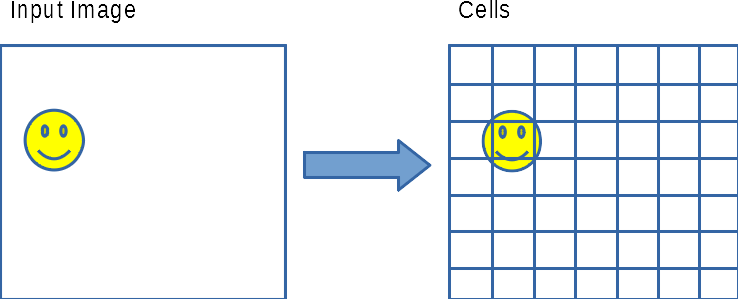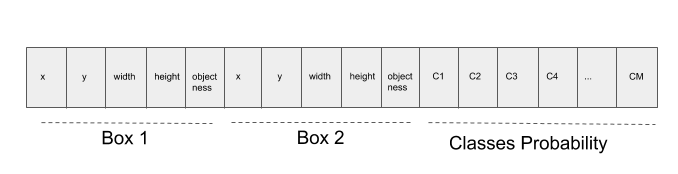# Building Head/Face Detection System <1. Concept>

This post is the first part of a collection of post described in https://alvissalim.com/abdullah-tracker/. This is a simple project that I want to build for my kid. In this post, we will learn how to build a face detection system using the PyTorch framework. Instead of taking of the easy way to simply use an existing tool to train object detector, we will try to build a custom model and training script for the purpose of understanding deeply how such models work.

There are many alternative models that we can use for head detection. We will build our model based on one of the most popular object detection model, Yolo (You Only Look Once: Unified, Real-Time Object Detection), with slight modifications. You can find the paper describing this model here : https://pjreddie.com/media/files/papers/yolo_1.pdf

In this post, we will base our implementation from the original paper and try to implement a custom training script and build our own slightly modified model. I will assume that you have basic knowledge of Python and PyTorch. If you are not familiar with either of them yet, it may be a bit difficult to follow. Nevertheless, I will try to explain the steps in this post as intuitively as possible so that you can even skip the technical detail and get an overview of how this works if you wish.

## What are we trying to do?

We are going to build a system for detecting heads based on the Yolo object detection model. You may be wondering, why heads detection, not face detection? The main reason is just I want to do something different. Another reason is that face detection only detects visible faces, while head detection is more general in that even when the person is looking back, we can still detect the head. I just want to build such system and see how we can use such system.

### How will it work?

There are many different approaches to perform object detection in an image. However Yolo and other Single-Shot method have the advantage that only one pass of the input image is needed. Other prior approached mostly works in 2 stages where the first stage is to create ROI proposals and a second stage is needed to process each proposed ROIs. These 2 stages approaches makes detection quite slow. Furthermore, the running time will increase as the number of proposal ROIs increase. Single Shot methods skips this problem by simultaneously generating prediction of bounding boxes and object categories.

In the above illustration, a 2 stages detection is shown. Given an image as an input, the system will generate a set of candidate Region of Interests (ROIs) which serve as potential detection candidates. Each ROIs is then processed to determine to determine which object category it belongs to. In the above example, assume that there are 2 categories, head and background.

In single shot method, the model skips the region proposal stage and simultaneously predict the bounding boxes and the object category. This reduces the computation time significantly since, we don’t need to process each ROI separately trough a different network. Furthermore, the computation time does not depend on the number of objects to be detected.

## Transforming Image to Boxes

Lets start with the simple idea that neural network is simply a system which transform information from one form to another. Object detection can be viewed as a system which transforms an image into a collection of boxes.

The next question that arises naturally is what kind of boxes should it transform an image to? This where things get interesting. We want the boxes to describe where the objects of interests are. We describe each object with a single box around it, as well as which category the objects belongs to.

Since each object gets one box, another question arise, which is “how many boxes should be output by the system?” The number of boxes that the system should output changes depending on the number of objects. How can we design such neural network with varying number of outputs? We want the network to run the input in a single pass, so recurrent neural network is not the answer that we want.

One way to solve this problem of varying number of output boxes is to simply generate boxes as many as possible and filter out the boxes that are not relevant. This may seems counter intuitive at first since we want to reduce computation cost. However, if we design the neural network to reuse most of the computation results, it can be more efficient than multi stage systems.

The strategy is to structure the boxes in such a way that encourages reuse of computational resources. We will structure the boxes corresponding to cells organized as a 2 dimensional grid. The image below shows how the output cells are organized as a 2D array. The cells are not the bounding boxes themselves, but rather acts as reference for multiple bounding boxes.Input image is converted into output cells organized as a 2D array

Each cell is linked to several boxes where each box’s center is inside the cell. In the example below each cell corresponds to 2 boxes. The boxes can have different width and height.

By organizing the boxes this way we can simplify the neural network by simply making it output a 2D structure where each element is a cell and each cell contains information for several boxes. The bounding box in a certain cell can be represented by 4 numbers (x_offset, y_offset, width, height). If we have N boxes for each cell, each cell should output (N * 4) numbers.

You may think that we have finally solved the problem. However, simply stopping here will give us a system which only a huge number of boxes. The next question is then how can we know which boxes actually corresponds to the object of interest? That brings us to the next discussion…

## Filtering the boxes

Now that the know how output boxes should be arranged, how do we filter out the boxes that are not relevant? We need additional information that we can use as reference in filtering out the boxes. One simple way is by assigning a score for each box. We can call this score the objectness score of the box. We can calculate the objectness based on many different criteria if we wish, but we can also simply add additional output for each box. Each box is now described with 5 numbers instead of 4 numbers (x_offset, y_offset, width, height, objectness).

## What object is inside the box?

Our model can now be used to detect objects using boxes. However, we have missed one important question. If we are searching for one type of object, then there is nothing to worry. What if we want detect different types of object? How do we know what category the object inside the object belongs to? Although our model for detecting heads only and doesn’t care about other objects, we will discuss how to do it anyway.

Naturally, we can add even more information for each box. We can add class probability distribution information to each box. If there are M number of classes of objects, then we will add M numbers to box. These M numbers adds up to 1 and each number represents the probability that the object inside the box belongs to a certain class.

Adding the class distribution information for each box may work, but it requires the model to estimate a big amount of numbers. If the number of classes is small it may be acceptable. However, when he have a large number of classes, the number becomes overwhelming.

Can we make it simpler? If we assume that each cell corresponds only to 1 object class, then we can reduce the number of output by simply augmenting the class probability distribution for each cell instead of each box. Using this assumption, we can achieve a much simpler model. One example of the information inside a cell with 2 boxes with M classes is shown below.Information in a cell of 2 boxes and M classes

## Quick Recap

I would like to keep each post short. So we will do a quick recap of the ideas we have explored so far:

• We have specified the expected input and outputs of the neural network
• The neural network model should output information about boxes
• The boxes are organized and grouped within cells, where each cell corresponds to a certain location on the image
• The boxes are then filtered out using the objectness criteria predicted by the neural network
• The class of that the object belongs to is estimated using the class probability distribution that is output by the neural network

Now that we have the concepts and have good expectation of the neural network model, we will write a code which implements this model in the next post.